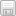# Write a SELECT statement that returns the total amount that customers paid for item_id = 5.

### OrcaleGiven the descriptions of tables above, complete the following tasks by providing the SQL statements in the space below. Be sure to provide your answers in the same order of the tasks.

1. Write a SELECT statement that determines the average cost of items by pub_id and returns the following columns: pub_id, number of items, average cost. Sort the result set by average cost in descending order.

2. Write a SELECT statement that returns the total amount that customers paid for item_id = 5. The paid amount for each order is calculated by paid_amount * quantity. (Hint: One item can be listed in several orders. Only total amount (one value) is returned as the output)

3. Write a SELECT statement that does INNER JOIN from the ORDERS table to the ITEMS table and returns the following columns: customer_id, ISBN, title, paid_amount, and quantity.

4. Write a SELECT statement that does LEFT JOIN from the PUBLISHERS table to the ITEMS table and returns the following columns: ISBN, title, name of publisher, and retail price.

5. Write a statement that modifies the cost and retails columns in the ITEMS table:

• Increase the cost by 5%
• Increase the retail by 10%